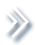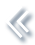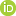####ArchivesReview Articles

## Quasi-Monte Carlo simulation of Brownian sheet with application to option pricing

Xinyu Song ,Yazhen WangPages 82-91 | Received 07 Mar. 2017, Accepted 17 May. 2017, Published online: 19 Jun. 2017,
• Abstract
• References
• Citations

ABSTRACT

Monte Carlo and quasi-Monte Carlo methods are widely used in scientific studies. As quasi-Monte Carlo simulations have advantage over ordinary Monte Carlo methods, this paper proposes a new quasi-Monte Carlo method to simulate Brownian sheet via its Karhunen–Loéve expansion. The proposed new approach allocates quasi-random sequences for the simulation of random components of the Karhunen–Loéve expansion by maximum reducing its variability. We apply the quasi-Monte Carlo approach to an option pricing problem for a class of interest rate models whose instantaneous forward rate driven by a different stochastic shock through Brownian sheet and we demonstrate the application with an empirical problem.

## References

1. Acworth, P., Broadie, M., & Glasserman, P. (1996). A comparison of some Monte Carlo and Quasi Monte Carlo techniques for option pricing. M. Carlo & Q.-M. Carlo Methods, Springer[Google Scholar]
2. Faure, H. (1982). Discrepence de suites associees a un systeme de numeration (en dimension s) Acta Arithmetica, 41, 337[Google Scholar]
3. Halton, J. H. (1960). On the efficiency of certain quasi-random sequences of points in evaluating multi-dimensional integrals. Numerische Mathematik, 2, 8490[Google Scholar]
4. Heath, D., Jarrow, R., & Morton, A. (1992). Bond pricing and the term structure of interest rates: A new methodology for contingent claims valuation. Econometrica, 60(1), 77105[Google Scholar]
5. Mallat, S. (2008). A wavelet tour of signal processing, third edition: The sparse way. Academic Press[Google Scholar]
6. Morokoff, W. J., & Caisch, R. E. (1995). Quasi-Monte Carlo integration. Journal of Computational Physics, 122, 218230[Google Scholar]
7. Niederreiter, H. (1973). Application of diophantine approximations to numerical integration. In C. F. Osgood, (Ed.), Diophantine approximation and its applications (pp. 129199). New York: Academic Press[Google Scholar]
8. Niederreiter, H. (1978). Quasi-Monte Carlo methods and pseudo-random numbers. Bulletin of the American Mathematical Society, 84, 9571041[Google Scholar]
9. Niederreiter, H. (1988). Low-discrepancy and low-dispersion sequences. Journal of Number Theory, 30, 5170[Google Scholar]
10. Niederreiter, H. (1988). Quasi-Monte Carlo methods for multidimensional numerical integration. In H. Brass & G. Hämmerlin (Eds.), Numerical integration III, international series of numerical math., (Vol. 85). Basel: Birkhäuser Verlag[Google Scholar]
11. Niederreiter, H. (1992). Random number generation and Quasi-Monte Carlo methods. Volume 63 of SIAM CBMS-NSF Regional Conference Series in Applied Mathematics. Philadelphia: SIAM[Google Scholar]
12. Santa-Clara, P., & Sornette, D. (2001). The dynamics of the forward interest rate curve with stochastic string shocks. Review of Financial Studies, 14(1), 149185[Google Scholar]
13. Sobol’, I. M. (1967). On the distribution of points in a cube and the approximation evaluation of integrals. USSR Computational Mathematics and Mathematical Physics, 7(4), 86112[Google Scholar]
14. Wang, X., & Fang, K.-T. (2003). Effective dimension and quasi-Monte Carlo integration. Journal of Complexity, 19, 101124[Google Scholar]